This package implements some graph layout algorithms that are not available in igraph.

A detailed introductory tutorial for graphlayouts and ggraph can be found here.

The package implements the following algorithms:

• Stress majorization (Paper)
• sparse stress (Paper)
• pivot MDS (Paper)
• dynamic layout for longitudinal data (Paper)
• a simple multilevel layout
• a layout algorithm using UMAP
• group based centrality and focus layouts which keeps groups of nodes close in the same range on the concentric circle

## Install

# dev version
remotes::install_github("schochastics/graphlayouts")

#CRAN
install.packages("graphlayouts")

## Stress Majorization: Connected Network

This example is a bit of a special case since it exploits some weird issues in igraph.

library(igraph)
library(ggraph)
library(graphlayouts)

set.seed(666)
pa <- sample_pa(1000,1,1,directed = F)

ggraph(pa,layout = "nicely")+
geom_node_point(col="black",size=0.3)+
theme_graph()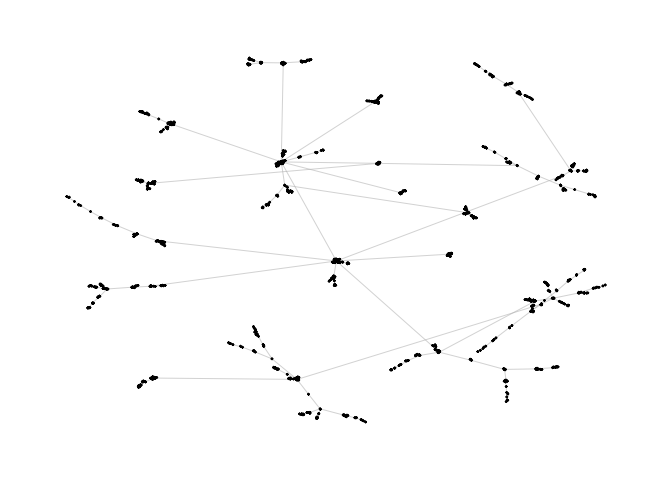ggraph(pa,layout="stress")+
geom_node_point(col="black",size=0.3)+
theme_graph()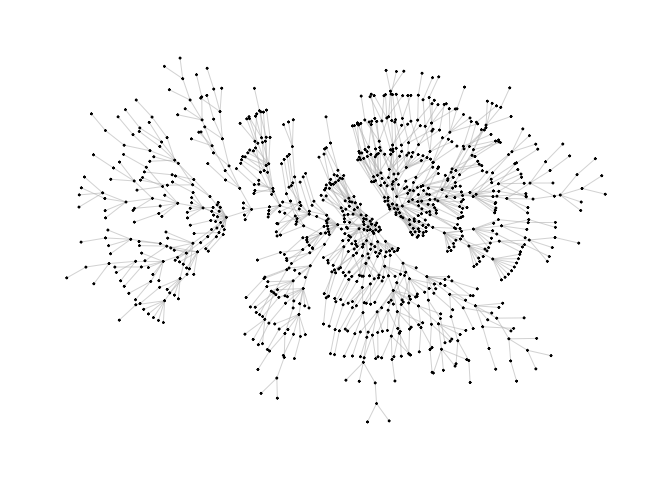## Stress Majorization: Unconnected Network

Stress majorization also works for networks with several components. It relies on a bin packing algorithm to efficiently put the components in a rectangle, rather than a circle.

set.seed(666)
g <- disjoint_union(
sample_pa(10,directed = F),
sample_pa(20,directed = F),
sample_pa(30,directed = F),
sample_pa(40,directed = F),
sample_pa(50,directed = F),
sample_pa(60,directed = F),
sample_pa(80,directed = F)
)

ggraph(g,layout = "nicely") +
geom_node_point() +
theme_graph()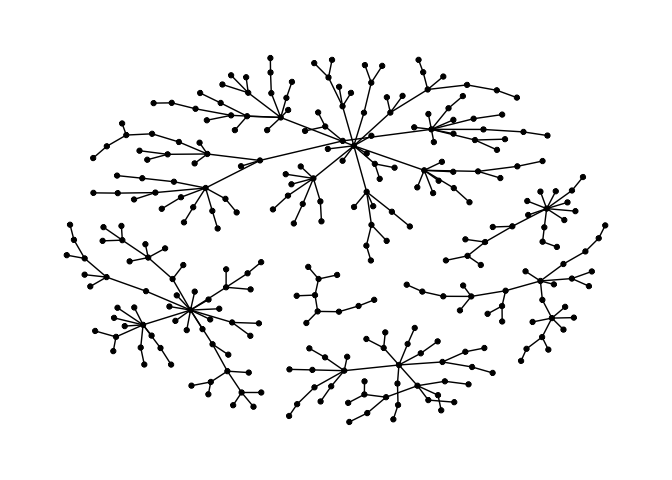ggraph(g, layout = "stress",bbox = 40) +
geom_node_point() +
theme_graph()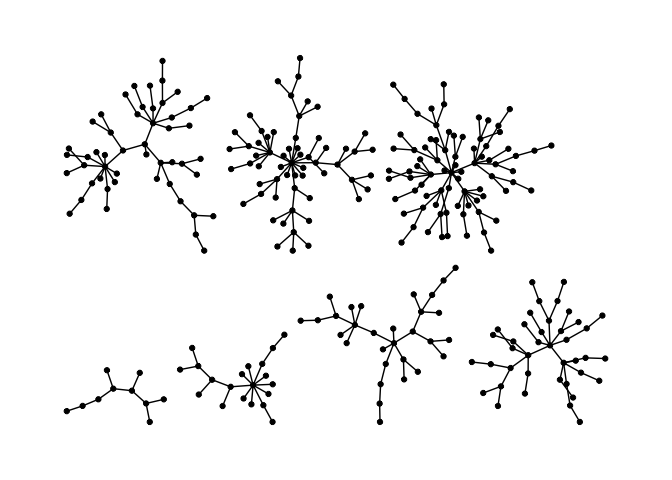## Backbone Layout

Backbone layouts are helpful for drawing hairballs.

set.seed(665)
#create network with a group structure
g <- sample_islands(9,40,0.4,15)
g <- simplify(g)
V(g)$grp <- as.character(rep(1:9,each=40)) ggraph(g,layout = "stress")+ geom_edge_link0(colour=rgb(0,0,0,0.5),width=0.1)+ geom_node_point(aes(col=grp))+ scale_color_brewer(palette = "Set1")+ theme_graph()+ theme(legend.position = "none")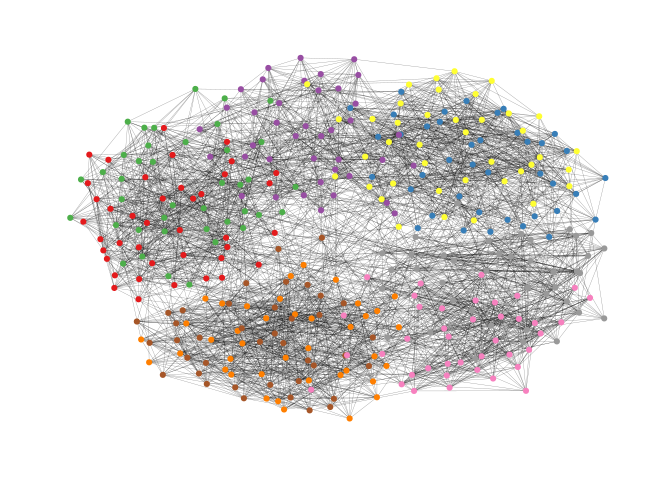The backbone layout helps to uncover potential group structures based on edge embeddedness and puts more emphasis on this structure in the layout. To use the function, you need to install the package oaqc install.packages("oaqc") bb <- layout_as_backbone(g,keep=0.4) E(g)$col <- F
E(g)$col[bb$backbone] <- T

ggraph(g,layout="manual",x=bb$xy[,1],y=bb$xy[,2])+
geom_node_point(aes(col=grp))+
scale_color_brewer(palette = "Set1")+
scale_edge_color_manual(values=c(rgb(0,0,0,0.3),rgb(0,0,0,1)))+
theme_graph()+
theme(legend.position = "none")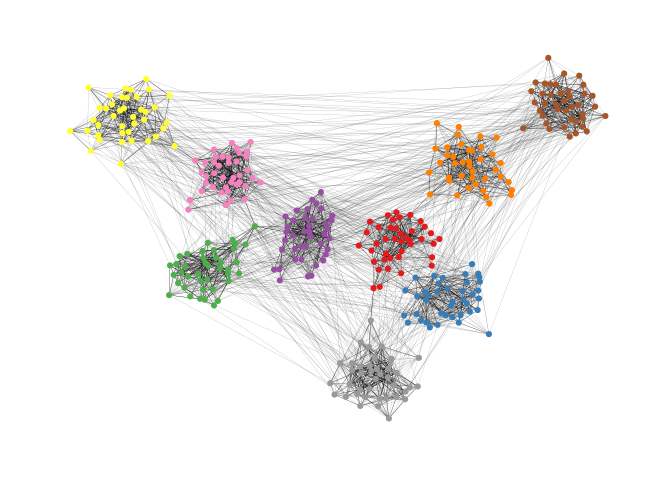## Radial Layout with Focal Node

The function layout_with_focus() creates a radial layout around a focal node. All nodes with the same distance from the focal node are on the same circle.

library(igraphdata)
library(patchwork)
data("karate")

p1 <- ggraph(karate,layout = "focus",focus = 1) +
draw_circle(use = "focus",max.circle = 3)+
geom_node_point(aes(fill=as.factor(Faction)),size=2,shape=21)+
theme_graph()+
theme(legend.position = "none")+
coord_fixed()+
labs(title= "Focus on Mr. Hi")

p2 <- ggraph(karate,layout = "focus",focus = 34) +
draw_circle(use = "focus",max.circle = 4)+
geom_node_point(aes(fill=as.factor(Faction)),size=2,shape=21)+
theme_graph()+
theme(legend.position = "none")+
coord_fixed()+
labs(title= "Focus on John A.")

p1+p2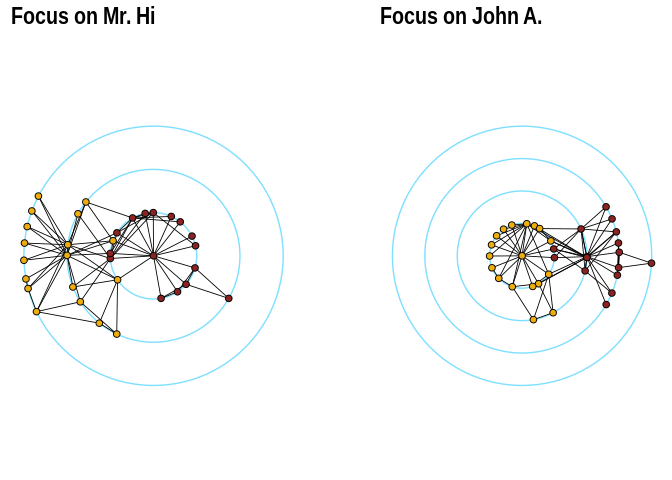The function layout_with_centrality creates a radial layout around the node with the highest centrality value. The further outside a node is, the more peripheral it is.

library(igraphdata)
library(patchwork)
data("karate")

bc <- betweenness(karate)
p1 <- ggraph(karate,layout = "centrality", centrality = bc, tseq = seq(0,1,0.15)) +
draw_circle(use = "cent") +
annotate_circle(bc,format="",pos="bottom") +
geom_node_point(aes(fill=as.factor(Faction)),size=2,shape=21)+
theme_graph()+
theme(legend.position = "none")+
coord_fixed()+
labs(title="betweenness centrality")

cc <- closeness(karate)
p2 <- ggraph(karate,layout = "centrality", centrality = cc, tseq = seq(0,1,0.2)) +
draw_circle(use = "cent") +
annotate_circle(cc,format="scientific",pos="bottom") +
geom_node_point(aes(fill=as.factor(Faction)),size=2,shape=21)+
theme_graph()+
theme(legend.position = "none")+
coord_fixed()+
labs(title="closeness centrality")

p1+p2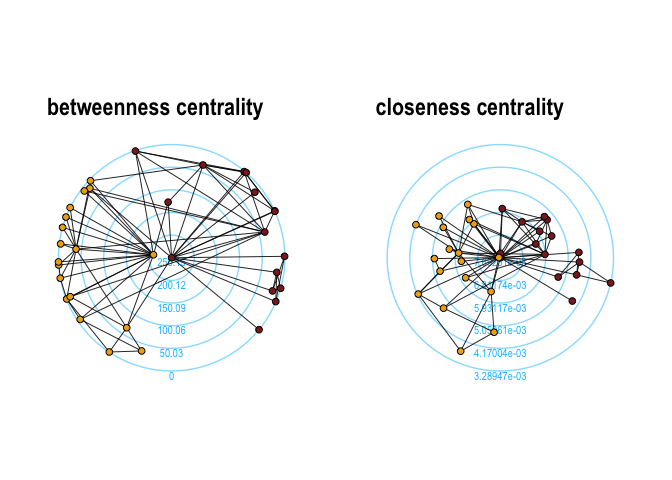## Large graphs

graphlayouts implements two algorithms for visualizing large networks (<100k nodes). layout_with_pmds() is similar to layout_with_mds() but performs the multidimensional scaling only with a small number of pivot nodes. Usually, 50-100 are enough to obtain similar results to the full MDS.

layout_with_sparse_stress() performs stress majorization only with a small number of pivots (~50-100). The runtime performance is inferior to pivotMDS but the quality is far superior.

A comparison of runtimes and layout quality can be found in the wiki
tl;dr: both layout algorithms appear to be faster than the fastest igraph algorithm layout_with_drl().

Below are two examples of layouts generated for large graphs using layout_with_sparse_stress()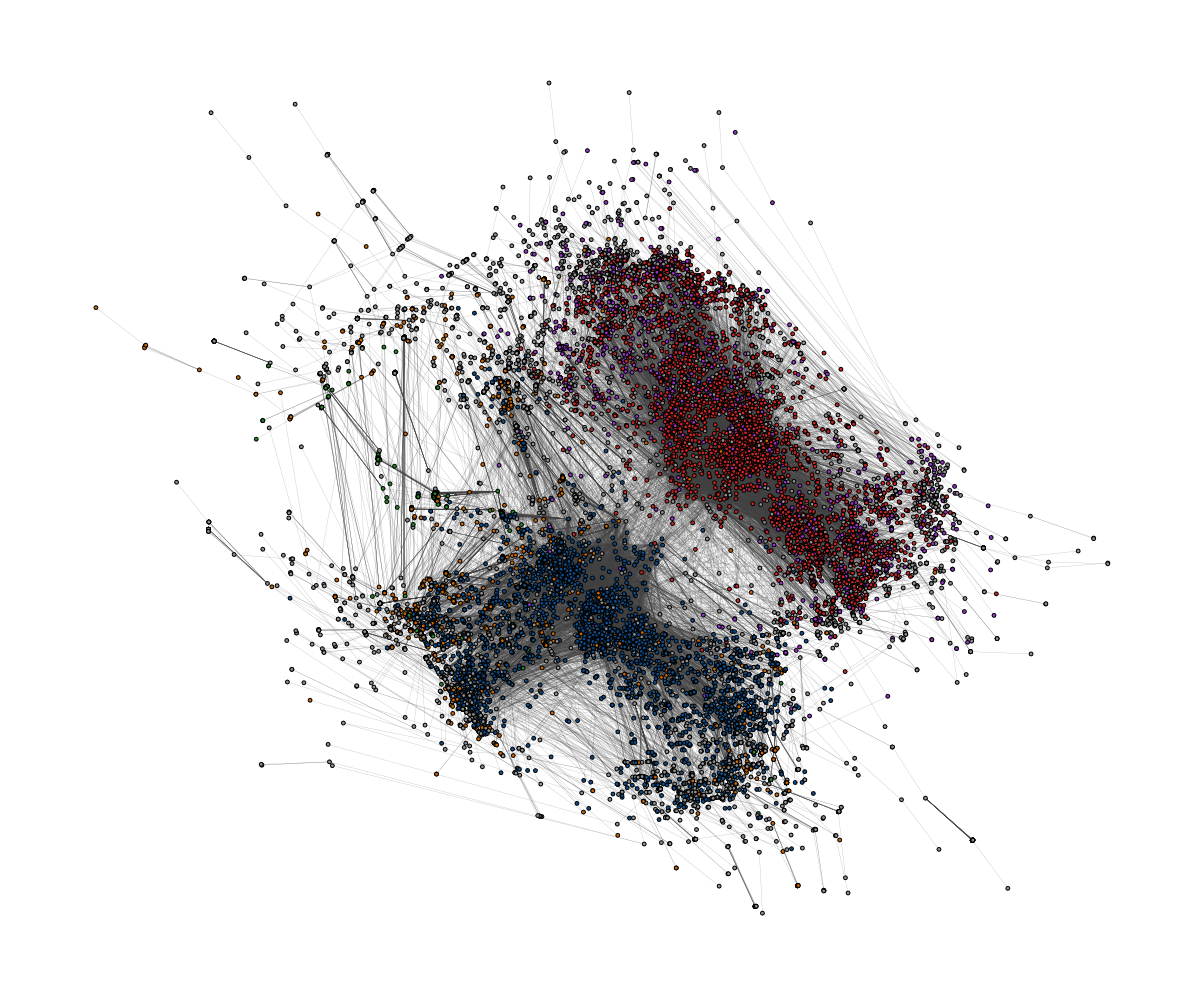A retweet network with 18k nodes and 61k edges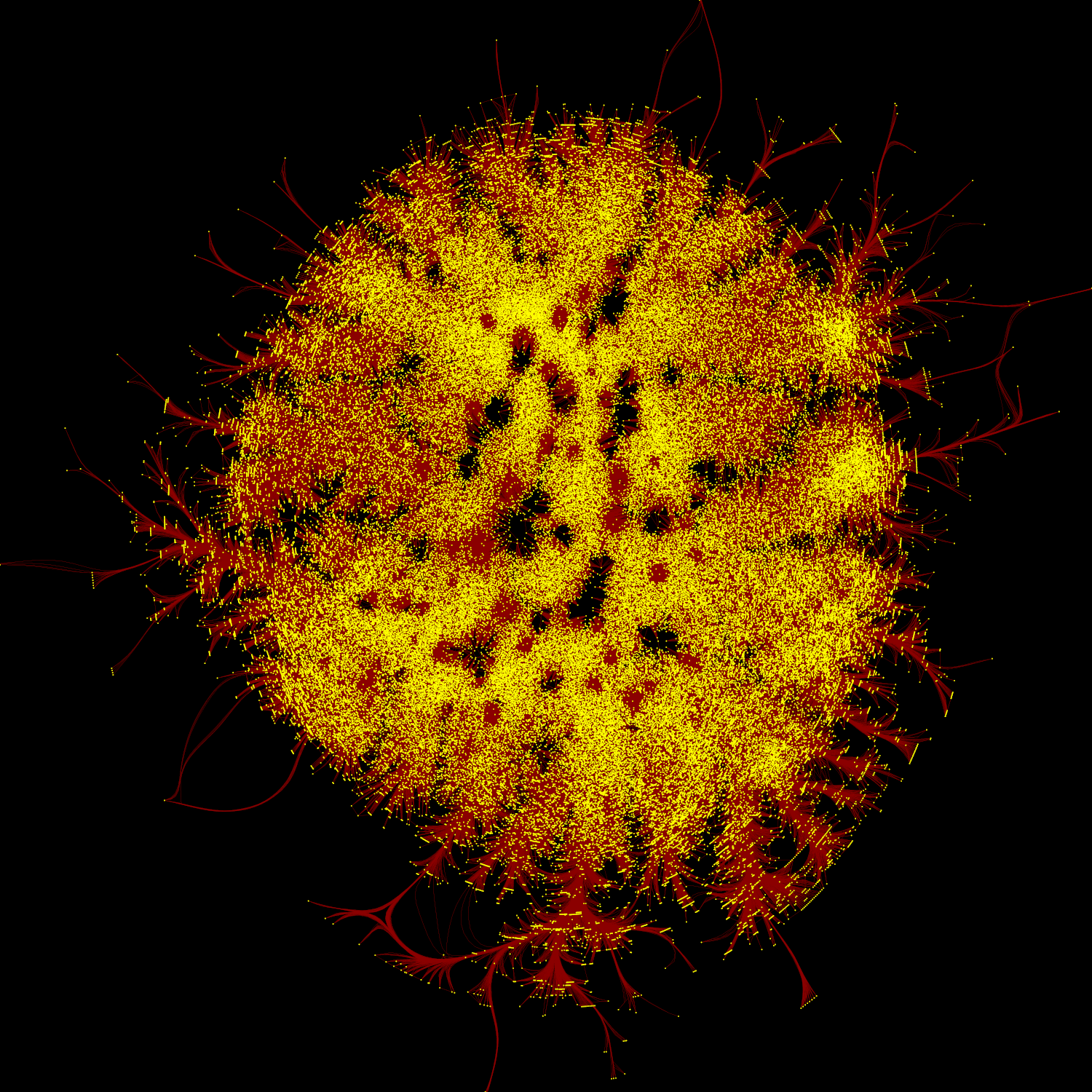A network of football players with 165K nodes and 6M edges.

## dynamic layouts

layout_as_dynamic() allows you to visualize snapshots of longitudinal network data. Nodes are anchored with a reference layout and only moved slightly in each wave depending on deleted/added edges. In this way, it is easy to track down specific nodes throughout time. Use patchwork to put the individual plots next to each other.

#remotes::install_github("schochastics/networkdata")
library(networkdata)
#longitudinal dataset of friendships in a school class
data("s50")

xy <- layout_as_dynamic(s50,alpha = 0.2)
pList <- vector("list",length(s50))

for(i in seq_along(s50)){
pList[[i]] <- ggraph(s50[[i]],layout="manual",x=xy[[i]][,1],y=xy[[i]][,2])+
geom_node_point(shape=21,aes(fill=as.factor(smoke)),size=3)+
geom_node_text(aes(label=1:50),repel = T)+
scale_fill_manual(values=c("forestgreen","grey25","firebrick"),
labels=c("no","occasional","regular"),
name = "smoking",
guide=ifelse(i!=2,"none","legend"))+
theme_graph()+
theme(legend.position="bottom")+
labs(title=paste0("Wave ",i))
}
wrap_plots(pList)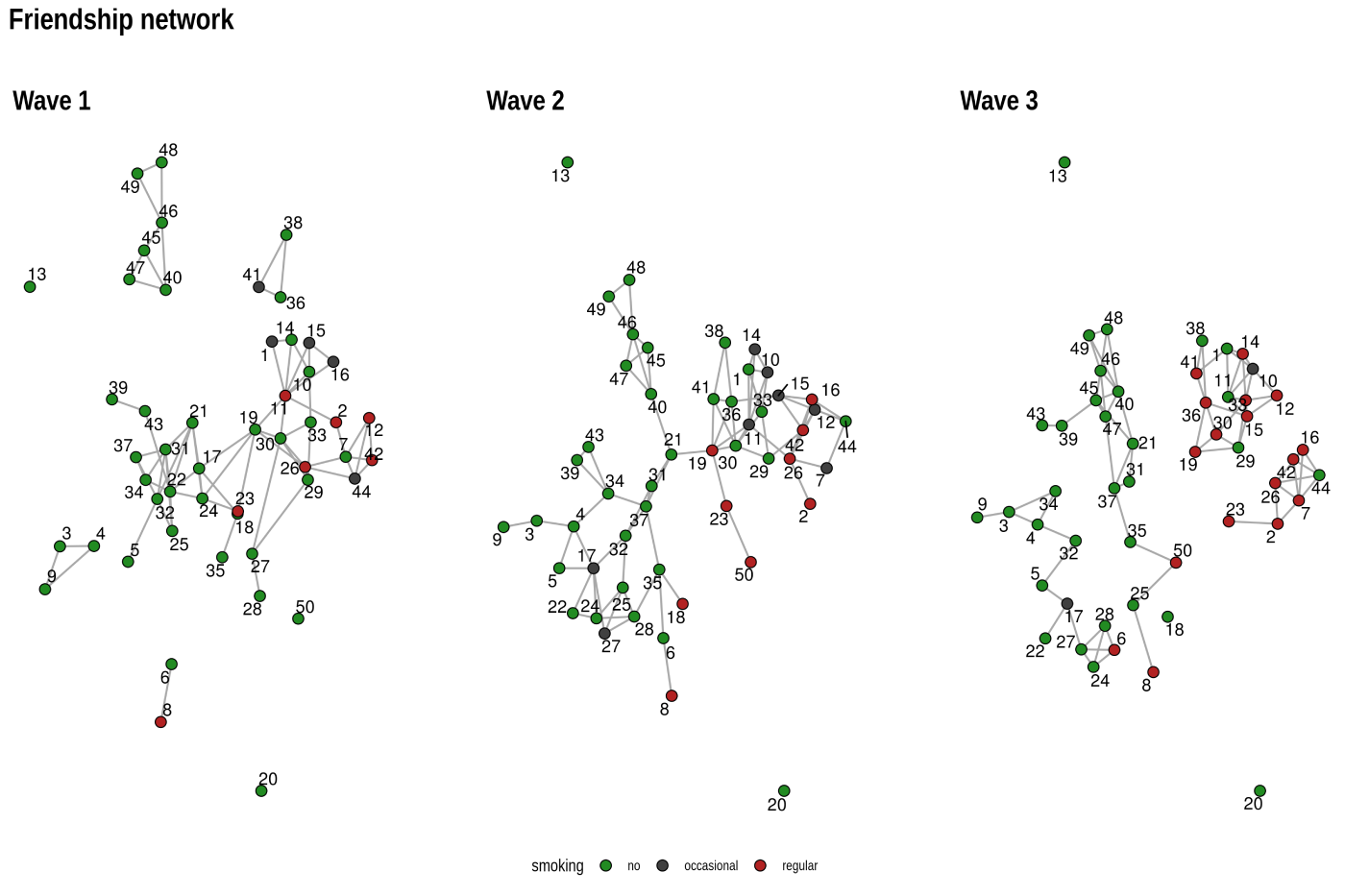## Layout manipulation

The functions layout_mirror() and layout_rotate() can be used to manipulate an existing layout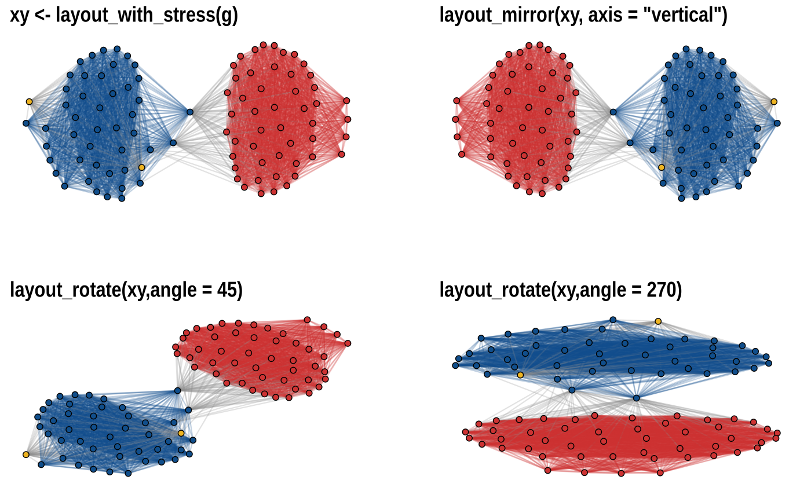# How to reach out?

### Where do I report bugs?

Simply open an issue on GitHub.

### How do I contribute to the package?

If you have an idea (but no code yet), open an issue on GitHub. If you want to contribute with a specific feature and have the code ready, fork the repository, add your code, and create a pull request.

### Do you need support?

The easiest way is to open an issue - this way, your question is also visible to others who may face similar problems.

### Code of Conduct

Please note that the graphlayouts project is released with a Contributor Code of Conduct. By contributing to this project, you agree to abide by its terms.